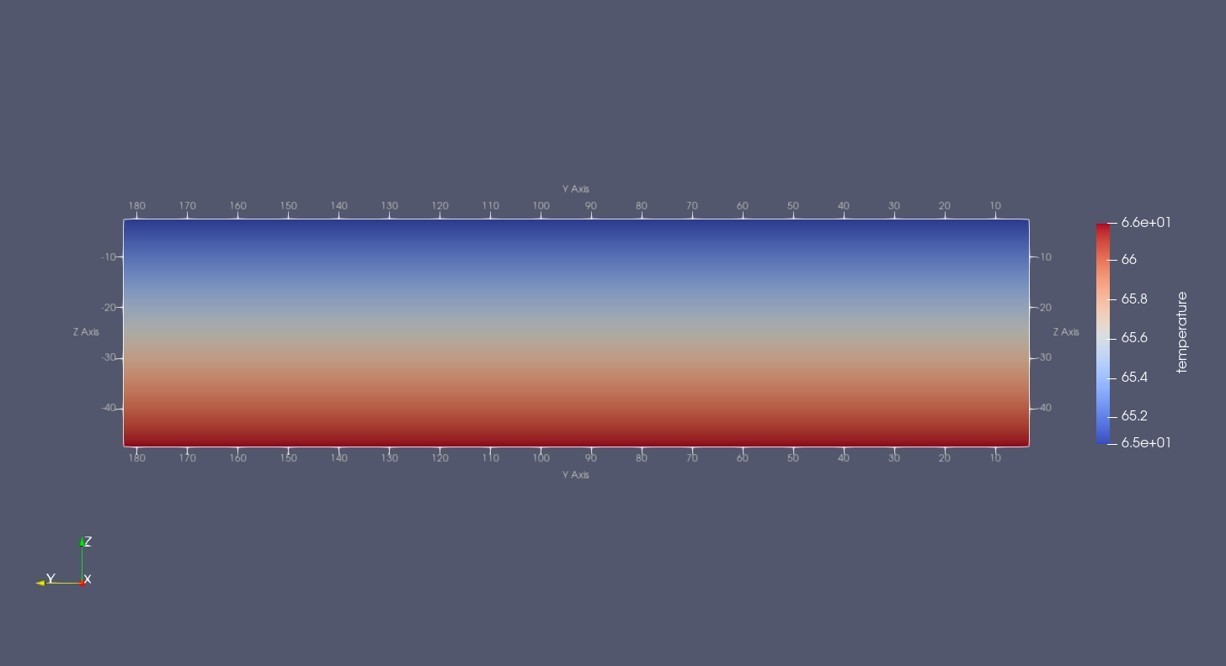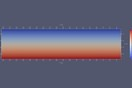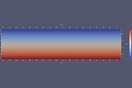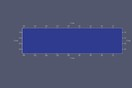0# Problem with visualizing material type in ParaViewHi every one,

I have run initialization simulation to obtain initial pressure and temperature conditions that follow hydrostatic pressure gradient and geothermal temperature gradient, respectively. The model has three material types; sand, well1, and well2. I have read the "INFILE" using Python and exported it to an unstructured VTK file and visualized it with ParaView. I could see the pressure and temperature results, however I couldn't see the material type. This is the code that I used to read the input file and export it to an unstructured VTK file.

import numpy
import toughio

points = numpy.array([v["center"] for v in parameters["elements"].values()])
incons = numpy.array([v["values"] for v in parameters["initial_conditions"].values()])

mesh = toughio.meshmaker.triangulate(points)
mesh.write("INFILE.vtu")

6replies Oldest first
• Oldest first
• Popular•You also have to export material data (which are stored for each element under the key "material"):

```rocks = numpy.array([v["material"] for v in parameters["elements"].values()])